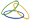##CADACUS, INC.

SOLUTIONS FOR SYSPRO™

As you are looking for Solutions to your SYSPRO™ needs, there may be other choices, but ask them if they have been working exclusively with SYSPRO™ since 1991. Ask them if they use the same NetExpress development system and tools SYSPRO uses. Very few other companies can say yes to both answers, but we can!

Providing quality solutions to the SYSPRO™ user community since 1991!

# Scripting for EDI - Multiple Insert Afters Multiple Times Method 1

#### Introduction

The script below is an example of how to perform multiple "Insert Afters" in a row multiple times.  Each function can only insert one segment.  Therefore the functions must be chained together to insert multiple segments.

This sample inserts two N1, N3 and N4 segments by having a separate function for every segment.

```

' Script to do multiple insert afters and multiple N1, N3 and N4 segmets - Method one

Function XXX()   ' Replace XXX with the segment you need to insert the segments after
'  Setup to call the function "Insert_N1" to insert the first N1 segment
EDI.InsertAfterFunction = "Insert_N1"  ' Tell the system to call 'Insert_N1' instead of the default 'InsertAfter' function
EDI.InsertAfter = True
End Function

Function Insert_N1()
EDI.InsertSegment = "N1"
EDI.Element(1) = "Qualifier"
EDI.Element(2) = "Customer Name"
EDI.Element(3) = "Location Qualifier"
EDI.Element(4) = "Location Code"

' After inserting the N1 segment, the following tells the system to insert another segment
' using the function "Insert_N3"

EDI.InsertAfterFunction = "Insert_N3"
EDI.InsertAfter = True
End Function

Function Insert_N3()
EDI.InsertSegment = "N3"
EDI.Element(1) = "Address Line 1"
EDI.Element(2) = "Address line 2"

' After inserting the N3 segment, the following tells the system to insert another segment
' using the function "Insert_N4"

EDI.InsertAfterFunction = "Insert_N4"
EDI.InsertAfter = True
End Function

Function Insert_N4()
EDI.InsertSegment ="N4"
EDI.Element(1) = "City"
EDI.Element(2) = "State"
EDI.Element(3) = "Postal Code"
EDI.Element(4) = "Country Code"

' After inserting the N4 segment, the following tells the system to insert another segment
' using the function "Insert_2nd_N1". This starts the 2nd set of N1, N3, N4 segments

EDI.InsertAfterFunction = "Insert_2nd_N1"
EDI.InsertAfter = True
End Function

Function Insert_2nd_N1()
EDI.InsertSegment = "N1"
EDI.Element(1) = "2nd Qualifier"
EDI.Element(2) = "2nd Customer Name"
EDI.Element(3) = "2nd Location Qualifier"
EDI.Element(4) = "2nd Location Code"

' After inserting the 2nd N1 segment, the following tells the system to insert another N3 segment
' using the function "Insert_2nd_N3"

EDI.InsertAfterFunction = "Insert_2nd_N3"
EDI.InsertAfter = True
End Function

Function Insert_2nd_N3()
EDI.InsertSegment = "N3"
EDI.Element(1) = "2nd Address Line 1"
EDI.Element(2) = "2nd Address line 2"

' After inserting the 2nd N3 segment, the following tells the system to insert another N4 segment
' using the function "Insert_2nd_N4"

EDI.InsertAfterFunction = "Insert_2nd_N4"
EDI.InsertAfter = True
End Function

Function Insert_2nd_N4()
EDI.InsertSegment ="N4"
EDI.Element(1) = "2nd City"
EDI.Element(2) = "2nd State"
EDI.Element(3) = "2nd Postal Code"
EDI.Element(4) = "2nd Country Code"
End Function```

### Current Releases

SYSPRO™ :  April 4, 2021
Handheld :  April 4, 2021